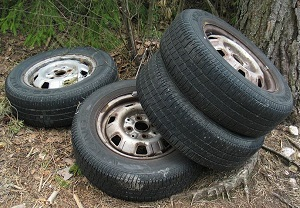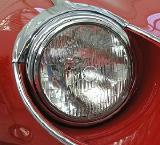# Limiting Reagents

When there is not enough of one reactant in a chemical reaction, the reaction stops abruptly. To figure out the amount of product produced, it must be determined reactant will limit the chemical reaction (the limiting reagent) and which reactant is in excess (the excess reagent). One way of finding the limiting reagent is by calculating the amount of product that can be formed by each reactant; the one that produces less product is the limiting reagent.

## Introduction

4 Tires + 2 Headlights = 1 Car+=Figure 1: The synthesis reaction of making a car. Images used from Wikipedia with permission.

The initial condition is that there must be 4 tires to 2 headlights. The reactants must thus occur in that ratio; otherwise, one will limit the reaction. There are 20 tires and 14 headlights, so there are two ways of looking at this problem. For 20 tires, 10 headlights are required, whereas for 14 headlights, 28 tires are required. Because there are not enough tires (20 tires is less than the 28 required), tires are the limiting “reactant.”

The limiting reagent is the reactant that is completely used up in a reaction, and thus determines when the reaction stops. From the reaction stoichiometry, the exact amount of reactant needed to react with another element can be calculated. If the reactants are not mixed in the correct stoichiometric proportions (as indicated by the balanced chemical equation), then one of the reactants will be entirely consumed while another will be left over. The limiting reagent is the one that is totally consumed; it limits the reaction from continuing because there is none left to react with the in-excess reactant.

There are two ways to determine the limiting reagent. One method is to find and compare the mole ratio of the reactants used in the reaction (approach 1). Another way is to calculate the grams of products produced from the given quantities of reactants; the reactant that produces the smallest amount of product is the limiting reagent (approach 2).

How to Find the Limiting Reagent: Approach 1

Find the limiting reagent by looking at the number of moles of each reactant.

1. Determine the balanced chemical equation for the chemical reaction.
2. Convert all given information into moles (most likely, through the use of molar mass as a conversion factor).
3. Calculate the mole ratio from the given information. Compare the calculated ratio to the actual ratio.
4. Use the amount of limiting reactant to calculate the amount of product produced.
5. If necessary, calculate how much is left in excess of the non-limiting reagent.

How to Find the Limiting Reagent: Approach 2

Find the limiting reagent by calculating and comparing the amount of product each reactant will produce.

1. Balance the chemical equation for the chemical reaction.
2. Convert the given information into moles.
3. Use stoichiometry for each individual reactant to find the mass of product produced.
4. The reactant that produces a lesser amount of product is the limiting reagent.
5. The reactant that produces a larger amount of product is the excess reagent.
6. To find the amount of remaining excess reactant, subtract the mass of excess reagent consumed from the total mass of excess reagent given.